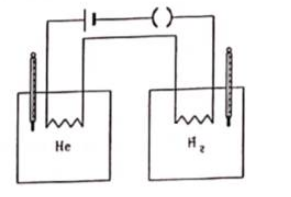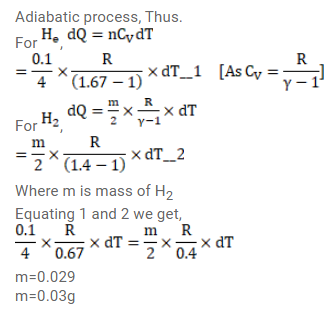# Figure shows two vessels with adiabatic walls,`
Question:

Figure shows two vessels with adiabatic walls, one containing $0.1 \mathrm{~g}$ of helium $(\gamma=1.67) \mathrm{M}=4 \mathrm{~g} / \mathrm{mol})$ and the other containing some amount of hydrogen $(\gamma=1.4, \mathrm{M}=2 \mathrm{~g} / \mathrm{mol})$. Initially, the temperatures of the two gases are equal. The gases are electrically heated for some time during which equal amounts of heat are given to the two gases. It is found that the temperatures rise through the same amount in the two vessels. Calculate the mass of hydrogen.Solution: